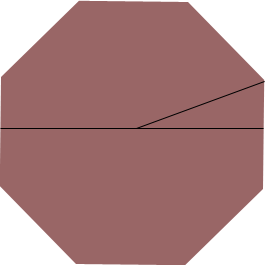Subject: the circle describing an octagon Name: Dave Who are you: Other (All) if the distance between two parallel sides of an octagon is 350 cm, what is the diameter of the circle that contains this octagon (ie. a circle that touches all eight corners)? Hi Dave. The diameter of the circle is twice its radius, so let's find the radius first. If you draw an octagon and join the midpoints of two opposite sides with a line, you'll have the line that represents the 350 cm in your question. This line must go through the center of the octagon/circle due to symmetry. So half of that 350 cm is the distance from a midpoint of a side of the octagon to the center. Now draw a line from the center of the circle to one of the endpoints of one of the sides you joined. This makes a small triangle.If you did this for every side's midpoint and every endpoint, you'd have sixteen spokes. You should see that by symmetry, each of the triangles is the same size and they are all right-angle triangles. The angle around a whole circle is 360 degrees, so the angle of a single triangle (the angle at the center, that is) is 1/16 of that (22.5 degrees). Each of the spokes that goes to an endpoint (in other words, to a corner of the octagon) goes from the center of the circle to the circle circumscribing the octagon, so it is the radius. So you now have a right triangle where you know the length of one side (half of 350 cm) and one other angle (22.5 degrees). The hypotenuse of the triangle is the radius you want to find, so if you now use the cosine function, you can find that radius. Cos A = adjacent / hypotenuse so radius = hypotenuse = (350 cm / 2) / cos (22.5) Double that is the diameter you are seeking. Hope this helps, Stephen La Rocque.